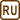## Electron microscope

Electron microscope can distinguish objects of scales around ten times bigger than the wavelength of used electrons. One microscope uses electrons accelerated to 10 keV. What is the smallest structure the microscope can distinguish?

• #### Hint

How are the energy of a particle and the wavelength of the corresponding de Broglie wave related?

• #### Solution

The relation between the kinetic energy of the particle E and wavelength  λ corresponding to de Broglie wave is given by formula

$\lambda=\frac{h}{\sqrt{2mE}}\,,$

where m is the mass of the particle and h is the Planck’s constant.

We use the mass of an electron m = 9.11·10−31 kg. We obtain

$\lambda=\frac{6.626\,\cdot\,10^{-34}}{\sqrt{2\,\cdot\,9.11\,\cdot\,10^{-31}\,\cdot\,1.602\,\cdot\,10^{-15}}}\ \mbox{m}\,=\frac{6.626}{\sqrt{2\,\cdot\,9.11\,\cdot\,1.602}}\ 10^{-11}\ \mbox{m}\,\dot{=}\,1.2\,\cdot\,10^{-11}\ \mbox{m}\,\dot{=}\, 0.01\ \mbox{nm}\,.$

Therefore the resolution power of the microscope is at least approximately tenths of a nanometer which are the units called ångström.